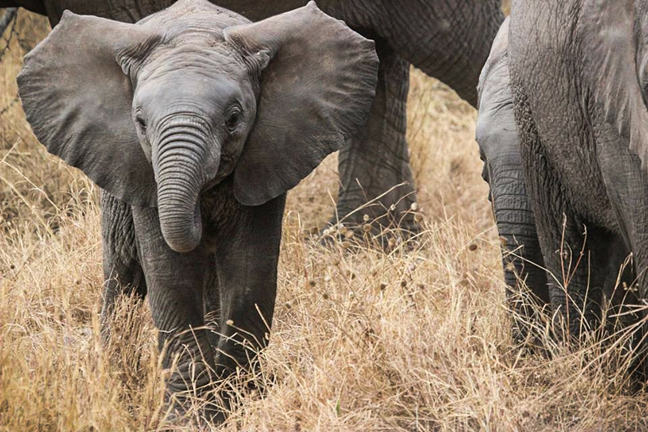## Want to keep learning?

This content is taken from the UNSW Sydney's online course, Maths for Humans: Linear, Quadratic & Inverse Relations. Join the course to learn more.
4.17

## UNSW SydneyA scaled down elephant

# Scaling laws in biology

It has been observed that the heartrate and metabolic rate per cell is less for large animals, and greater for small animals. All well and good, but having some equations relating precise variables gives us a lot more power to understand, make predictions and perhaps change our lifestyle accordingly!

In this step we find out how these quantities are related mathematically.

## Lifespans

In recent decades biologists have started to appreciate that many phenomenon scale with body size according to mathematical laws – but these are not linear, quadratic or inverse relationships. They are a little bit more subtle: they are power laws.

A cat has about $$\normalsize{100}$$ times the mass of a mouse, and lives about three times longer. Now observe that

$\Large{100^\frac{1}{4} \approx 3.}$

We say that lifespan scales (roughly) as mass to the one-quarter power:

$\Large{\operatorname{lifespan} \approx \operatorname{constant} \times \operatorname{mass}^\frac{1}{4}.}$

Q1 (E): Pick an animal from the table of lifespans in the previous question. Estimate its mass and try to determine the constant in this equation. Put your value for the constant in the discussion section.

Recall that when we raise a number to the $$\normalsize{\frac{1}{2}}$$ power, that is like taking a square root. So raising a number to the power $$\normalsize{\frac{1}{4}}$$ is taking the square root of the square root. This can only be done approximately in general.Q2: Assuming the correctness of this law, what relation should there be between the lifespan of a cat and that of an elephant? Hint: a cat weighs about $$\normalsize{4}$$ kgs, while an elephant weights about $$\normalsize{4000}$$ kgs.

## Heartrates

Here is another interesting difference that depends on the size of an animal: bigger animals have slower heart rates, while smaller animals have faster heartrates. In fact heartrate has been observed to scale as mass to the minus one-quarter power:

$\Large{\operatorname{heartrate}\approx\operatorname{constant} \times \operatorname{mass}^{-\frac{1}{4}}}.$

If I live to a hundred years, and each year my heart beats $$\normalsize{12}$$ million times, then the total number of times my heart has beaten throughout my entire life will be the product of these numbers, namely $$\normalsize{1.2}$$ billion.

We can see this from the units involved:

$\Large{\operatorname{time}\times\frac{\operatorname{beats}}{\operatorname{time}} = \operatorname{beats} }.$

So if we put the two equations for lifespans and heartrates together, the exponents of $$\normalsize{\frac{1}{4}}$$ and $$\normalsize{\frac{-1}{4}}$$ will cancel, and a remarkable new law becomes evident. We find that

$\Large{\operatorname{lifespan}\times\operatorname{heartrate}\approx\operatorname{constant} }.$

In other words, lifespan and heartrate are inversely proportional. And the total number of heartbeats is roughly constant across species: at roughly a billion. What a remarkable world we live in!

A2. Since the elephant weighs about $$\normalsize{4000}$$ kgs and a cat about $$\normalsize{4}$$ kgs, the elephant is about $$\normalsize{1000}$$ times as heavy. Now $$\normalsize{1000^{1/4} \approx 5.6}$$, so we expect that the elephant’s lifespan is about $$\normalsize{5.6}$$ times the lifespan of a cat. Clearly we ought to consider natural lifespans here. Probably a domestic cat lives longer than a wild cat, and certainly elephants in zoos live shorter lives than elephants in the wild. In fact the oldest recorded wild elephant lived to $$\normalsize{86}$$ years in Asia, and elephants in their natural state live longer than any other land mammal, aside from man.